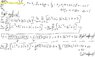# Riemann sum where n = 3 for both left and right endpoint estimates

• s3a
In summary, Vela attempted to solve a problem involving integrating a function f(x) from x=-1 to x=4 using Riemann sums with n=3, twice. However, she incorrectly summed the constant terms. Homework Statement Compute the integral that is highlighted in MyWork.jpg using Riemann sums using left and right endpoints.

## Homework Statement

Compute the integral that is highlighted in MyWork.jpg using Riemann sums using left and right endpoints.

## Homework Equations

##x_i* = a + i Δx##
##*x_i = a + i Δx - Δx##
##Σ_{i=1}^{n} i = n(n+1)/2##
##Σ_{i=1}^{n} i^2 = n(n+1)(2n+1)/6##

## The Attempt at a Solution

My attempt is attached as MyWork.jpg. I've done this problem several times and, I cannot figure out what I am doing wrong. I would really appreciate it if someone could point out what I am doing wrong!

#### Attachments

•MyWork.jpg
47.2 KB · Views: 407
Last edited:
First, review this post, especially item #4.

Was it the indices to the summations that you wanted me to review? (I just added them.)

If you're talking about ##*x_i## equation, it could be wrong since I derived that myself using ##x_i*## and a drawing of the rectangles and the curve but, I don't know what I did wrong (assuming that I did, in fact, do something wrong).

If it's something else, tell me and I will review it.

Hello, again.

Is what I did still not what you asked?

I'm not clear why you spend so much time writing general formulas when the problem is very specific. You are to integrate $f(x)= 2x^2+ 2x+ 3$, from x= 1 to x= 4, using "Riemann sums" with n= 3, twice, once using left endpoints, then using right endpoints. Okay, the distance from 1 to 4 is 4- 1= 3, as you say, and dividing into 3 equal parts means each part has length $\Delta x= 1$.

The left endpoints then are x= 1, 2, and 3. The Riemann sum is $f(1)\Delta x+ f(2)\Delta x+ f(3)\Delta x= (f(1)+ f(2)+ f(3))\Delta x$.

The right endpoints are 2, 3, and 4. The Riemann sum is $f(2)\Delta x+ f(3)\Delta x+ f(4)\Delta x= (f(2)+ f(3)+ f(4))\Delta x$.

That's pretty straightforward arithmetic.

You just summed the constant terms incorrectly. For example, you had
$$\sum_{i=1}^3 (2i^2+2i+3) = 2\sum_{i=1}^3 i^2 + 2 \sum_{i=1}^3 i + 3\sum_{i=1}^3 1 = 2(14) + 2(6) + 3 \sum_{i=1}^3 1.$$ You got the right answer for the first two sums, but you messed up the last summation. You simply added 3 instead of 3×3=9.

Edit: Sorry, I double-posted.

HallsofIvy, I was definitely over-complicating things!

Vela, I didn't just dump the picture out of laziness; the image is clear since it was created digitally and, whoever checked my thread out had to open it anyway to see my work so, I thought it was just more convenient for whoever checks my thread out to see the question and work all in one place. Rethinking it now, though, people probably want to see the problem at a quick glance before even considering to bother answering so, I guess I will put the question in both places next time.

Thank you both!